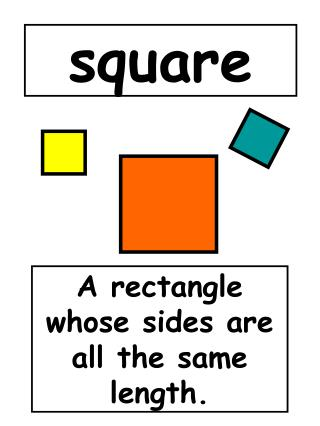DownloadDownload Presentationsquare

square

Download Presentationsquare

- - - - - - - - - - - - - - - - - - - - - - - - - - - E N D - - - - - - - - - - - - - - - - - - - - - - - - - - -
Presentation Transcript

1. square A rectangle whose sides are all the same length.

2. square array A rectangular array with the same number of rows as columns.

3. 1 square number 4 9 2 16 A number that is the product of a whole number multiplied by itself; a whole number to the second power. 35 = 35 x 35 = 1225

4. square of a number The product of number and itself.

5. square pyramid A pyramid with a square base.

6. square root of a number 320= 2*2*2*2*2*2*5 320= 4*4*4*5 or320=16*4*5 √320=8√5 The square root of a number n is a number which, when multiplied by itself, results in the number n.

7. square units 7 square units The units used to measure area.

8. 587 = 500 + 60 + 7 standard notation expanded notation standard notation The most familiar way of representing whole numbers, integers, and decimals by writing digits in specified place.

9. standard unit A uniform unit of measure.

10. step graph A graph that looks like steps.

11. stem-and-leaf plot A display of data in which digits with larger place values are named as stems, and digits with smaller place values are names as leaves.

12. straightedge A tool used to draw a line segment.

13. 7 - 3 minuend subtraction subtrahend difference 4 A math operation based on taking away one quantity from another or decreasing quantity.

14. 7 - 3 minuend subtrahend subtrahend difference 4 The number being subtracted.

15. addends sum sum 14 + 15 + 6 = 35 The answer to an adding problem.

16. supplementary angels Two angels whose measures total 180 . o

17. survey Collection data for graphing and analysis.

18. symmetry The property of exact balance in a figure; having the same size and shape across a dividing line.

19. symmetry The matching of two halves of a shape.

20. tally marks 11 5 10 Marks used to keep track of a count.

21. tally chart Tally charts are used to organize and display data. A tally chart is a table that shows how many times each value appears.

22. tangent Intersecting at exactly one point.

23. template A pattern used as a guide in making something accurately.

24. 59 270 tens The place-value position equal to ten times the unit value.

25. term In an algebraic expression or equation, a number or a product of a number and one or more variables.

26. terminating decimal .333… 1 3 = .30 Decimals that continue a pattern without end.

27. tessellation An arrangement of closed shapes that covers a surface completely without overlaps or gaps.

28. tetrahedron A polyhedron with four faces.

29. theorem A mathematical statement that can be proven to be true (or sometimes, a statement that is proposed and needs to be proven).

30. 3-dimensional (3-D) Objects with thickness,length and width.

31. 3-dimensional (3-D) Objects that are not completely within a single flat surface.

32. tile A shape used in a tessellation. If only one shape is repeated in a tessellation, the tessellation is called a same-tile tessellation.

33. tiling An arrangement of closed shapes that covers a surface completely without overlaps or gaps.

34. time line A number line showing years, days,and events in a sequence.

35. tool kit A bag containing things needed in math.

36. top-heavy fraction A fraction that names a number greater than or equal to 1; a fraction whose numerator is equal to or greater than its denominator.

37. trade-first subtraction Subtraction procedure in which all necessary trades are done before any subtractions are carried out.

38. trapezoid A quadrilateral that has one pair of parallel sides. No two sides need to be the same length.

39. translation The motion of “sliding” an object or picture along a straight line.

40. transparent mirror A mirror with a front and back face that has a recessed edge used for drawing lines of symmetry.

41. triangle A 3-sided polygon.

42. triangular prism A prism whose bases are triangles.

43. triangular pyramid A pyramid whose bases (faces) are triangles.

44. One trillion written in number form is 1,000,000,000,000 in exponential form 10 trillion A digit(s) followed by 12 whole-number places. 12

45. triple Three times an amount.

46. turn Rotation.

47. 2 x 3 3 x 2 6 + 3 3 + 6 turn-around facts 234 x 5 5 x 234 143 + 23 23 + 143 A pair of add or multiplication facts in which the order of the addends or the factors is reversed.

48. 3 and 5 are twin primes, because they are separated by the composite number 4. twin primes Two prime numbers that are separated by just one composite number.

49. 2-dimensional (2-D) Objects completely within a flat surface; objects with length and width, but no thickness.

50. 24 children unit 27 ducks 1 kg 3 o’clock 30 cents A label, descriptive word, or unit of measure used to put a number in context.